Scatter Plots: What they are and how to make the most of them

by    Aug 21, 2020 7:03 pm
In :
from mpl_toolkits.axes_grid1 import AxesGrid
from matplotlib.colors import ListedColormap
from matplotlib.pyplot import figure
import matplotlib.pyplot as plt
from vega_datasets import data
import seaborn as sns
import pandas as pd
import numpy as np
import matplotlib
import random

In :
%matplotlib inline
random.seed(126)

sns.set_style("darkgrid", {"axes.facecolor": ".9"})
sns.set_context("talk", font_scale=.7)

Introduction¶

In this article, we are going to show you how to create scatter plots using Python's seaborn package and the movies dataset available in the vega_datasets package. To avoid visual clutter, only a subset of the data is used to create the visualizations.

In :
df = data.movies()

#Select columns
cols = ["Title", "MPAA Rating", "Source", "Major Genre",
"US Gross", "US DVD Sales", "Production Budget",
"Running Time min", "IMDB Rating", "IMDB Votes"]
df = df[cols]

#Drop any row with missing values
df.dropna(axis = 0,
how = 'any',
inplace = True)

You can see the first five rows of the resulting dataset below.

In :

Out:
Title MPAA Rating Source Major Genre US Gross US DVD Sales Production Budget Running Time min IMDB Rating IMDB Votes
1064 12 Rounds PG-13 Original Screenplay Action 12234694.0 8283859.0 20000000.0 108.0 5.4 8914.0
1068 1408 PG-13 Based on Book/Short Story Horror 71985628.0 49668544.0 22500000.0 102.0 6.9 72913.0
1074 2012 PG-13 Original Screenplay Action 166112167.0 50736023.0 200000000.0 158.0 6.2 396.0
1082 28 Weeks Later R Original Screenplay Horror 28638916.0 24422887.0 15000000.0 91.0 7.1 69558.0
1090 300 R Based on Comic/Graphic Novel Action 210614939.0 261252400.0 60000000.0 117.0 7.8 235508.0

1. What is a Scatter Plot?¶

A scatter plot visualizes the relationship between a pair of numerical variables. The value of one variable is plotted on the x-axis, and the value of the second is plotted on the y-axis; in this way, the values of the variables serve as coordinates.

The plot below shows the relationship between the runtime of a movie and the number of votes that the movie received on IMDB. The coordinates of an outlier in the dataset are shown as a text annotation

In :
#Variables needed for p1
x_coord = df[df["IMDB Votes"] == y_coord]["Running Time min"].max()

#Text for annotation
text = '(' + str(x_coord) + ', ' + str(y_coord) + ')'

In :
figure(figsize=(10,8))

#Create the scatter plot
p1 = sns.scatterplot(x="Running Time min",
data=df);

#Revise the axis labels
p1.set(xlabel='Runtime (minutes)');

#Plot the coordinates of an outlier in the data
p1.text(x_coord + 1, y_coord,
text,
horizontalalignment='left',
size='medium',
color='black');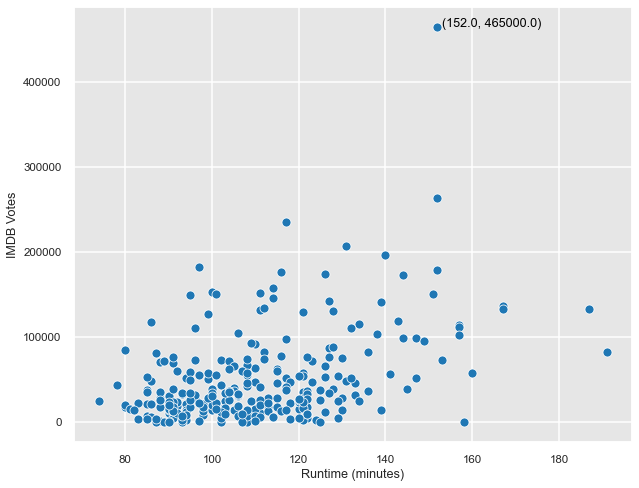2. Scatter Plots in Exploratory Data Analysis¶

Because of their ability to show the relationship between a pair of variables, scatter plots are fundamental for exploratory data analysis. Pairwise scatter plots can be quickly generated to provide a high-level view of the trends present in a dataset.

The chart below shows a scatter plot for each pair of numerical variables in the dataset, including the relationship of each variable with itself along the diagonal! Although it may seem arbitrary, the plot of the relationship of a variable with itself can still be meaningful: for some of the graphs along the diagonal, it is clear that the points are more dense in certain places (typically the lower-left corner); however, this information is better visualized by a different chart type, such as a histogram or a density plot.

In :
#Determine which columns contain numerical variables
numerical_cols = [col for col in df.columns if df[col].dtype in ['int64', 'float64']]

In :
#Create the scatter plot
p2 = sns.pairplot(df,
x_vars=numerical_cols,
y_vars=numerical_cols,
diag_kind=None) #diag_kind can be changed to see a different type of graph along the diagonal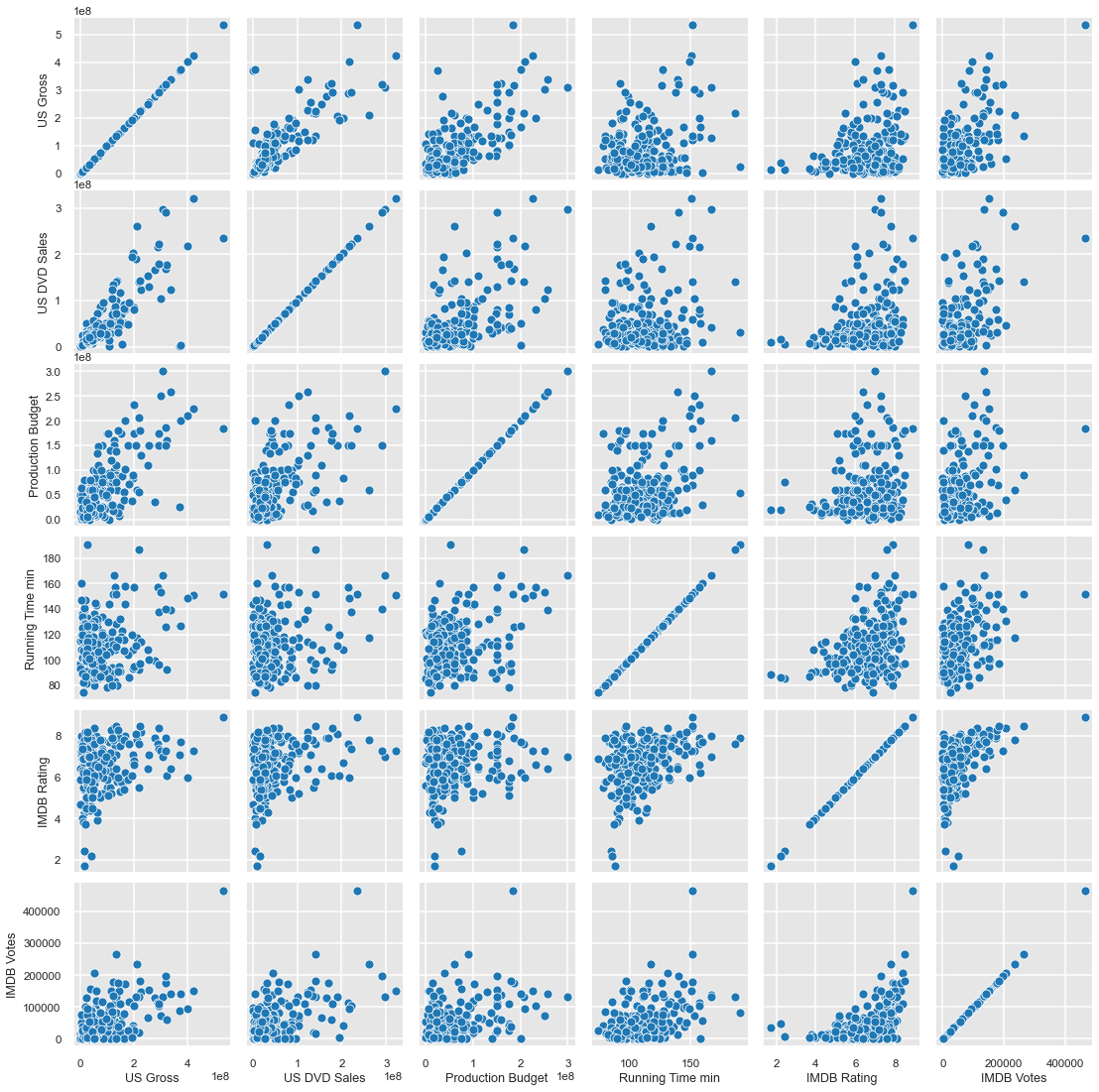3. Scatter Plots in Regression Analysis¶

With a scatter plot, you are able to see how two variables are related, but if you want to confirm the type of relationship present, it is useful to model the relationship. In certain cases, such as regression analysis, the variables on the x- and y-axis are referred to as the independent variable and the dependent variable, respectively.

In the graph below, US DVD Sales is plotted against US Gross, and the best fit line is shown. These variables seem to have a linear relationship, but as US Gross increases, its ability to predict US DVD Sales may decrease, as highlighted by the confidence interval (shown as the shaded region).

In :
figure(figsize=(10,8))

#Create the scatter plot
#Note that a higher confidence interval (ci) will result in a larger shaded area
p3 = sns.regplot(x="US Gross",
y="US DVD Sales",
ci=99,
data=df)

#Change the x- and y-axis labels
p3.set(xlabel='US Gross (independent variable)',
ylabel='US DVD Sales (dependent variable)');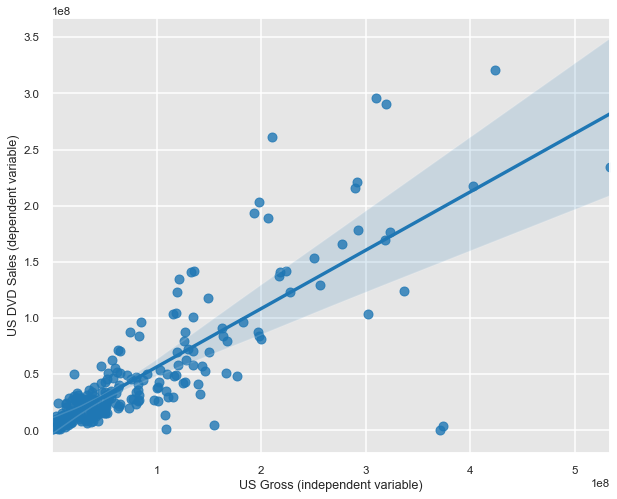4. Using Color to Display a Third Dimension¶

a. Categorical Variables¶

Color can be used in a scatter plot to consider a third dimension, and it is most often used to display a categorical variable. If the third variable has a strong relationship with the other two variables, then the points may form clusters of the same color.

In the graph below, the MPAA Rating is given by the color of the point. It appears as if movies rated for more mature audiences (i.e., R and PG-13) receive more IMDB votes but result in lower US DVD Sales than movies for younger audiences (with ratings of G and PG).

In :
figure(figsize=(10,8))

#Create the scatter plot
p4a_i = sns.scatterplot("US DVD Sales",
hue="MPAA Rating",
hue_order=["G", "PG", "PG-13", "R"], #To show the ratings in order
data=df);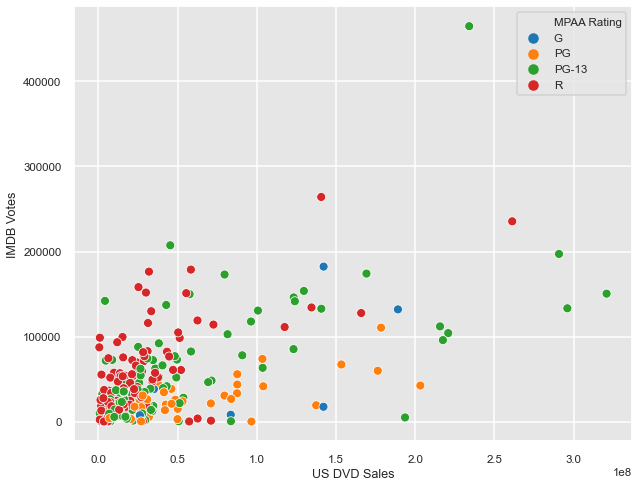b. Numerical Variables¶

Although less common, it is also possible to use color to show a numerical variable as the third dimension of a scatter plot; to do so, a color gradient is used.

In the graph below, the color is used to show how each movie compares to the dataset average in terms of US Gross. It is clear that movies that grossed more in the United States tend to receive more votes and higher ratings on IMDB.

In :
# Create a normalized version of US Gross
df["US Gross (norm)"] = (df["US Gross"] - df["US Gross"].mean())/(df["US Gross"].std())

In :
#Source: https://www.thetopsites.net/article/50003503.shtml

def shiftedColorMap(cmap, start=0, midpoint=0.5, stop=1.0, name='shiftedcmap'):
cdict = {'red': [],
'green': [],
'blue': [],
'alpha': []}

reg_index = np.linspace(start, stop, 257)

shift_index = np.hstack([
np.linspace(0.0, midpoint, 128, endpoint=False),
np.linspace(midpoint, 1.0, 129, endpoint=True)])

for ri, si in zip(reg_index, shift_index):
r, g, b, a = cmap(ri)
cdict['red'].append((si, r, r))
cdict['green'].append((si, g, g))
cdict['blue'].append((si, b, b))
cdict['alpha'].append((si, a, a))

newcmap = matplotlib.colors.LinearSegmentedColormap(name, cdict)
plt.register_cmap(cmap=newcmap)

return newcmap

In :
#Create a gradient centered at 0
orig_cmap = ListedColormap(sns.color_palette("RdYlGn", 10).as_hex())
shifted_cmap = shiftedColorMap(orig_cmap, midpoint=0, name='shifted')

In :
figure(figsize=(10,8))

#Create the scatter plot
p4b = sns.scatterplot("IMDB Rating",
hue="US Gross (norm)",
data=df,
palette=shifted_cmap)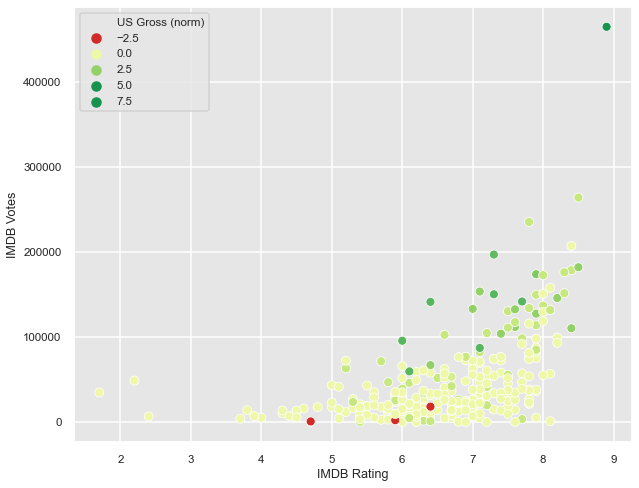5. Bubble Charts¶

In addition to color, size can be used to add a dimension to a scatter plot. When you use size to show a third dimension, the resulting graph is commonly referred to as a bubble chart.

The graph below shows the same variables as the graph above, but this time, US Gross is shown as the size. Again, the graph shows that movies that received more votes and higher scores tend to have higher values for US Gross.

In :
figure(figsize=(10,8))

#Create the scatter plot
p5 = sns.scatterplot("IMDB Rating",
size="US Gross",
sizes=(2, 200),
alpha=0.6,
data=df)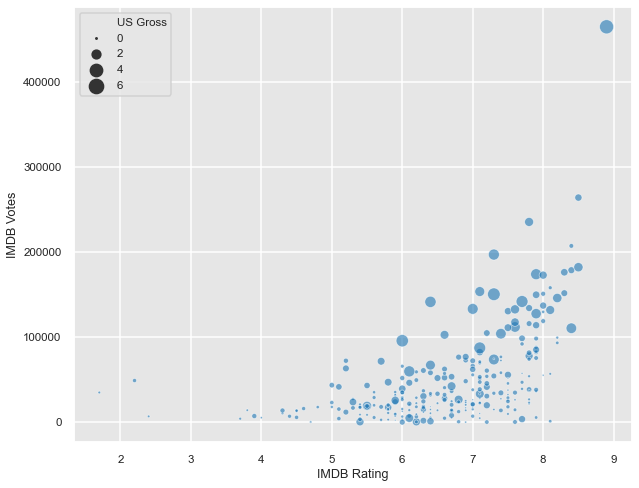Conclusion¶

Although scatter plots are simple charts, they can be very useful tools for exploring the relationships between variables.

Continue to make data-driven decisions.

Sign up for our email guides that contains relevant tips, software tricks, and news from the data world.

*We never spam you or sell your information.

* indicates required

<< Previous Post

"Protected: Wording Surveys Well Makes Them More Effective: Part 4"

"Pie Charts"